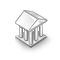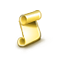Interesting script?
So post a link to it -
let others appraise it

You liked the script? Try it in the MetaTrader 5 terminal# IncMatrix - library for MetaTrader 5

Views:
3568
Rating:
Published:
2012.08.09 14:23

Class for working with matrices.

Matrix is created in one dimensional array, in sequence: elements of first row, second and so on. The last two elements represent the size of matrix: number of columns and rows.

Example:

```double m[]={1,2,3,
4,5,6,
2,3}; // Matrix of two rows and three columns.```

Class methods:

Method Description of method and parameters

void SetSize(
double& aA[],
int aRows,
int aCols
)

Sets the size of matrix aA. aRows - number of rows, aCols - number of columns.

void SetValue(
double& aA[],
int aRow,
int aCol,
double aValue
)

Sets the value (Value) of matrix (aA) element located in row aRow, column aCol.

int GetSize(
double& aA[],
int& aRows,
int& aCols
)

Returns the number of matrix aA elements. By reference returns: aRows - number of rows, aCols - number of columns.

int GetRows(
double& aA[]
)

Returns the number of rows in matrix aA.

int GetCols(
double& aA[]
)

Returns the number of columns in matrix aA.

double GetValue(
double& aA[],
int aRow,
int aCol
)

Gets the value of element in matrix aA located in row aRow and column aCol.

void Copy(
double& aFrom[],
double& aTo[]
)

Copies matrix from array aFrom to array aTo.

double& aA[],
double& aB[]
)

Checks if two matrices match by size for addition (fully equivalent by height and width).

bool CheckForMult(
double& aA[],
double& aB[]
)

Checks if two matrices match by size for multiplication (number of columns in matrix aA equals the number of columns in matrix aB).

bool CheckIsSq(
double& aA[]
)

Checks if matrix is square.

double& aA[],
double aNum,
double& aR[]
)

Adds number aNum to matrix aA. Resulting matrix is returned by reference in array aR.

void MultNum(
double& aA[],
double aNum,
double& aR[]
)

Multiplies matrix aA by number aNum. Resulting matrix is returned by reference in array aR.

double& aA[],
double& aB[],
double& aAB[]
)

Adds matrix aA to matrix aB. Resulting matrix is returned by reference in array aAB.

void MultMx(
double& aA[],
double& aB[],
double& aAB[])

Multiplies matrix aA by matrix aB. Resulting matrix is returned by reference in array aAB.

void Transpose(
double& aA[],
double& aT[]
)

Transposes matrix aA. Transposed matrix is returned by reference in array aT.

double& aA[],
double& aAA[]
)

Gets cofactor matrix. aA - source matrix, aAA - cofactor (returned by reference).

bool Invert(
double& aA[],
double& aB[]
)

Returns the inverse matrix aR of matrix aA by reference. The method returns true if inverse matrix exists or false, if inverse matrix does not exist.

void Triangle(
double& aA[],
double& aT[]
)

Returns triangular matrix aT from matrix aA by reference.

void Minor(
double aA[],
int aRow,
int aCol,
double& aM[]
)

Gets the minor of matrix aA by row aRow and column aCol. Minor is returned by reference in array aM.

double MinorDef(
double& aA[],
int aRow,
int aCol
)

Returns the determinant value of the matrix aA minor by row aRow and column aCol.

void MinorDefMx(
double& aA[],
double& aM[]
)

Gets minors matrix (matrix with values of the minors determinants). aA - source matrix, aM - matrix with minors determinants (returned by reference).

double Def(
double& aA[]
)

Returns the determinant value of matrix aA.

int Rank(
double& aA[]
)

Returns rank of matrix aA.

int RankDRC(
double& aA[],
int& aRow,
int& aCol
)

Returns rank of matrix aA and by reference returns:

• aDef - the determinant value,
• aRow - row of minor with determinant not equal to 0
• aCol - column of minor with determinant not equal to 0

void CopyCol(
double& aFrom[],
double& aTo[],
int aFromCol,
int aToCol,
double& aR[]
)

Copies column with index aFromCol from matrix aFrom to matrix aTo to column with index aToCol. Result is returned by reference in array aR.

void CopyRow(
double& aFrom[],
double& aTo[],
int aFromRow,
int aToRow,
double & aR[]
)

Copies row with index aFromRow from matrix aFrom to matrix aTo to row with index aToRow. Result is returned by reference in array aR.

void AppendCol(
double& aA[],
double& aC[],
double& aF[]
)

Extends matrix aA by adding column aC to it. Result is returned by reference in array aF.

void AppendRow(
double& aA[],
double& aR[],
double& aF[]
)

Extends matrix aA by adding row aR to it. Result is returned by reference in array aF.

bool SystemKramer(
double& aK[],
double& aY[],
double& aX[]
)

Solves system of linear equations using Cramer's rule.

• aK - coefficient matrix (square),
• aY - column of values,
• aX - row of results

bool SystemInverse(
double& aK[],
double& aY[],
double& aX[]
)

Solves system of linear equations using invertible matrix.

• aK - coefficient matrix (square),
• aY - column of values,
• aX - row of results

bool SystemGauss(
double& aK[],
double& aY[],
double& aX[]
)

Solves system of linear equations using Gaussian elimination.

• aK - coefficient matrix (square),
• aY - column of values,
• aX - row of results

int SystemCheck(
double& aK[],
double& aY[]
)

Checks system of equations.

• aK - coefficient matrix (square),
• aY - column of values.

Returned value:

• -1 - no solutions,
• 0 - one solution,
• 1 - infinite number of solutions

double& aA[],
string aCaption=""
)

Displays the entire matrix in one alert box.

• aA - matrix,
• aDigits - number of digits after decimal point,
• aCaption - message title

double& aA[],
string aCaption=""
)

Displays matrix in alert box line by line, rows displayed from bottom to top, then title, i.e. in alert box matrix is oriented normally: title at the top, then rows in order.

double& aA[],
)

Displays array of matrix as a string in alert box.

The sMatrix.mq4 script is an example of using this library to solve a system of linear equations using Cramer's rule, invertible matrix and Gaussian elimination.

Translated from Russian by MetaQuotes Software Corp.
Original code: https://www.mql5.com/ru/code/951Widescreenshooter

Script to create wide screenshots.Choppy Market Index

Indicator of market instability.GMACD

Two-Line MACD. This indicator is available by default in a great deal of trading platforms. But it cannot be found in Metatrader 5.ResultRetcodeDescription

The function that decrypts the code of the trade operation result for the functions OrderSend() and OrderCheck().